# Dividends

This page explains how to work with dividends in the Binomial Option Pricing Calculator.

The calculator supports four types of underlying securities: stocks, indexes, currencies and futures. Dividends apply to the first two. In order to make the pricing models consider dividends, either Stock or Index must be selected in the Underlying Type dropdown box in cell C6 in the Main sheet.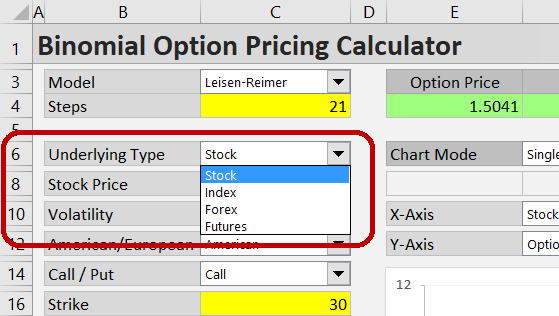For stocks, the calculator can work with either continuous or discrete dividends. You can select which one you prefer in the dropdown box in cell C28, labeled “Dividends As”.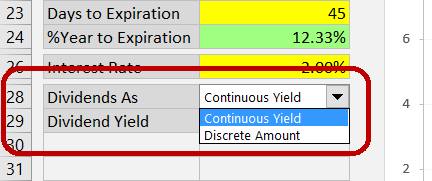You won’t see this dropdown box when Index is selected as underlying type, as the model always works with continuous dividends for index options. The treatment of continuous dividends in the calculations is the same for stock and index options. It is explained below.

## Continuous Dividends

When “Continuous Yield” is selected in the dropdown box in C28, the only dividend input you need to enter is the continuous dividend yield. Enter it in the yellow cell C29, labeled “Dividend Yield”.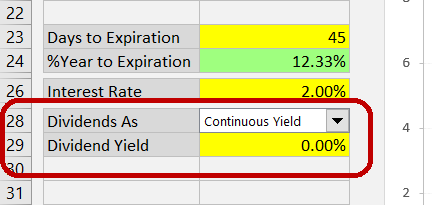The model assumes dividends are paid continuously, a common approach in financial models. It means there are an infinite number of infinitely small dividend payments. This is unrealistic – in the real world, dividends are paid as discrete amounts only one or a few times per year (quarterly for most US stocks).

However, in most cases the effect of this simplification on the resulting option price is not material. Exceptions include dividends which are particularly large (relative to underlying price) or due very soon. In such cases it can be more accurate to use the other format – enter the dividend as a discrete amount.

## Discrete Dividends

With discrete dividends, two inputs are required.

Firstly, you need to enter the dividend amount, per share (or generally in same units as underlying price and strike price), in the yellow cell C30, which is labeled “Dividend Amount”. (When continuous yield is selected in C28 or an underlying type different than Stock is selected in C6, the Dividend Amount cell will be grey and won’t work.)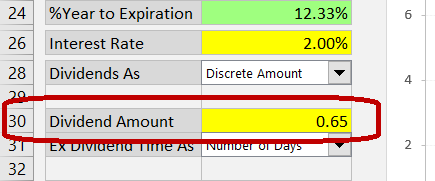Secondly, you need to specify when the stock will go ex dividend. It is the ex dividend date, not the dividend payment date. When a stock goes ex dividend, its price typically decreases (roughly by the amount of the dividend, not assuming other market moves). This is bad for call options (unlike stock holders, option holders won’t get the dividend, but the stock price decline applies to both). Conversely, lower underlying price is good for put option holders.

In the calculator, you can enter the ex dividend time in three different ways, just like the option’s time to expiration.

## Entering Time to Ex Dividend

First, select the preferred format in the dropdown box in cell C31 (“Ex Dividend Time As”).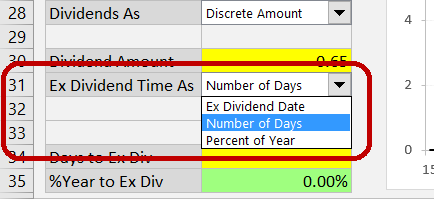If you have selected Ex Dividend Date format, enter the date (and optionally time) when the stock will go ex dividend in the yellow cells below (C32, C33).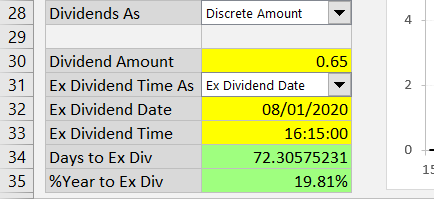If you have selected Number of Days, enter the number of days from now (the moment of valuation, usually today) to the moment the stock goes ex dividend. You can enter fractions of days too.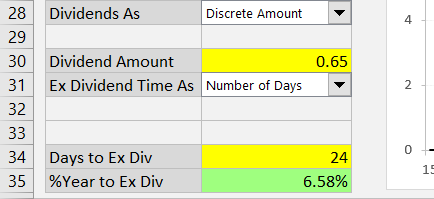If you have selected Percent of Year, enter the time between valuation and ex dividend as percent of year (number of days divided by 365).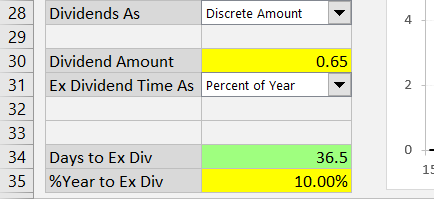## Time to Expiration vs. Ex Dividend Format

Most users will prefer to use the same format for time to expiration and time to ex dividend – for instance, enter both as number of days or both as dates and times. This is also the calculator’s default behavior – when you change the format for time to expiration, time to ex dividend will also switch to this format (converting the existing value).

You can choose to enter time to expiration and time to ex dividend in different formats. To do so, select the time to expiration format first (the dropdown box in C18) and then select the time to ex dividend format (C31).

There is one limitation (all other combinations work): You can’t use time to ex dividend as “Ex Dividend Date”, unless time to expiration is also set as dates and times (“Start and End” format).

The reason is simple: Unless time to expiration is entered as “Start and End” (valuation and expiration date), the calculator doesn’t know the valuation date and won’t be able to compute the time to ex dividend from ex dividend date. Therefore, when you try to switch the ex dividend format to “Ex Dividend Date” and time to expiration is set to something other than “Start and End”, you will see this message: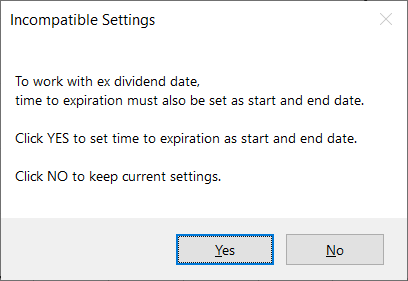Clicking Yes will set both time to expiration and ex dividend as dates; clicking No will keep the existing formats.

## Modeling Effects of Dividends

Like with other inputs, you can use the chart to model effects of dividends (dividend yield, dividend amount or ex dividend date) on option prices and Greeks – for detailed instructions see Working with the Chart.

For instance, the chart below shows how differences in dividend amount affect the relationship between stock price and option price.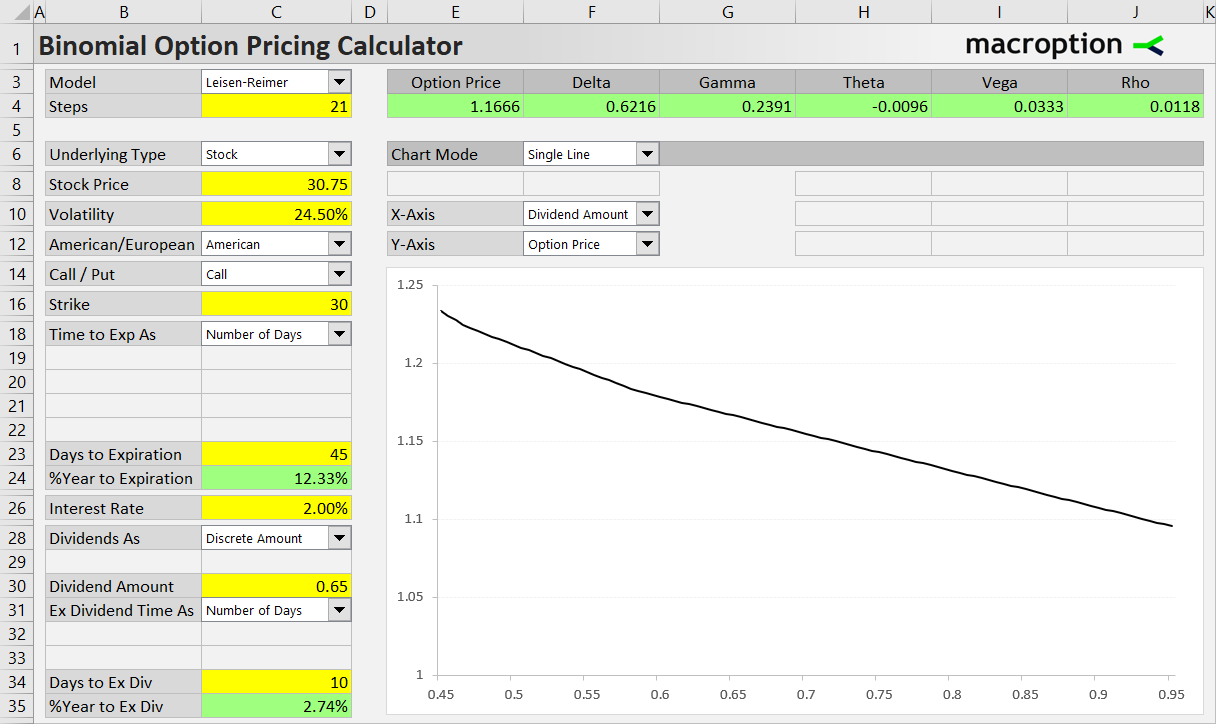Note: When working with discrete dividends, sometimes the chart may look a bit rugged, showing stairs rather than a smooth line. This is not an error. It is due to the way discrete dividends and binomial models (also discrete in nature) work together. If you want to see a smooth line, either increase the model’s number of steps or choose continuous dividends instead of discrete.

Have a question or feedback? It takes less than a minute.